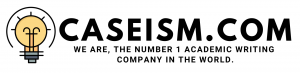# Carded Graphics, LLC Case Solution & Answer

## Carded Graphics, LLC Case StudyÂ Analysis

### Cost of Capital Analysis / Discount rate Calculations

Murry Pitts decided to use the financial data from comparable companies, using an appropriate discount factor and the traditional net present value method to evaluate the investments in the equipment. The 6% discount rate is calculated based on the information extracted from a comparable international packaging company. The calculations are shown in Exhibit 1 of the document.

### Marginal Cash Flow Analysis / Present Value of the Difference in Both Costs

According to the appendices, the marginal cash flow calculation after tax has been completed. The calculation period is 9 years, starting in 2009 and ending in 2017. Furthermore, the initial amount of capital expenditures was consolidated at the end of fiscal year 2009 and the CAPEX is \$ 700,000.

However, at the end of 2009; the company had the sales of \$ 14,100,514, which increased due to two average indices (i.e., 7.00% and 8.00%). Therefore, the percentage of profit earned was 7.50%, which was applied to Carded Graphics’ sales revenue.

Moreover, the cost of goods sold increased by 2.00%,which is derived from the information available in this case. As a result of which, the cost of goods was \$ 11,276,894, with an increase of 2.00%. Selling and administrative expenses also increased at the rate of 2.00%.

As a result, the company earned the last 9 years of interest and pre-tax income. The amount based on the additional treatment fee was obtained in this case, and \$ 22,500 fee increased by 2.00%.

At the same time, it also consolidated the working capital by deducting the current liabilities from the current assets, and where appropriate; applying a growth rate of 2.00%. The new letter opener has a turnover of \$ 120,000, and the cost savings are related to avoiding the new employees.

He also calculated the profit and loss based on the sales of the old paper printer; the loss was \$ 11,000. Interest expense is also calculated by applying a growth rate of 2% to the interest expenses for the financial year of 2009. The depreciation amount is also calculated by dividing the cost of the injection molding machine by the useful life span: 5 years, and deducting the remaining value from the cost of the tablet, between \$ 120,000 and \$ 700,000.

Therefore, the depreciation result is \$ 64,444. In addition to this, pre-tax operating cash flow was achieved for the period of 9 years. Pre-tax operating cash flow is expected to apply a tax rate of 30%, and it is assumed that the income tax expense for 2009 will be divided by the pre-tax profit.

The net present value is calculated by deducting the cost of saving new equipment; all costs must be incremental, which means that the new equipment is directly connected to each other. For this calculation, we assume a tax rate of 30%, an annual growth rate of savings of 3%, and an NPV of \$ 18,801,018for the new printer. The calculations are shown in Exhibit 2 of the document.